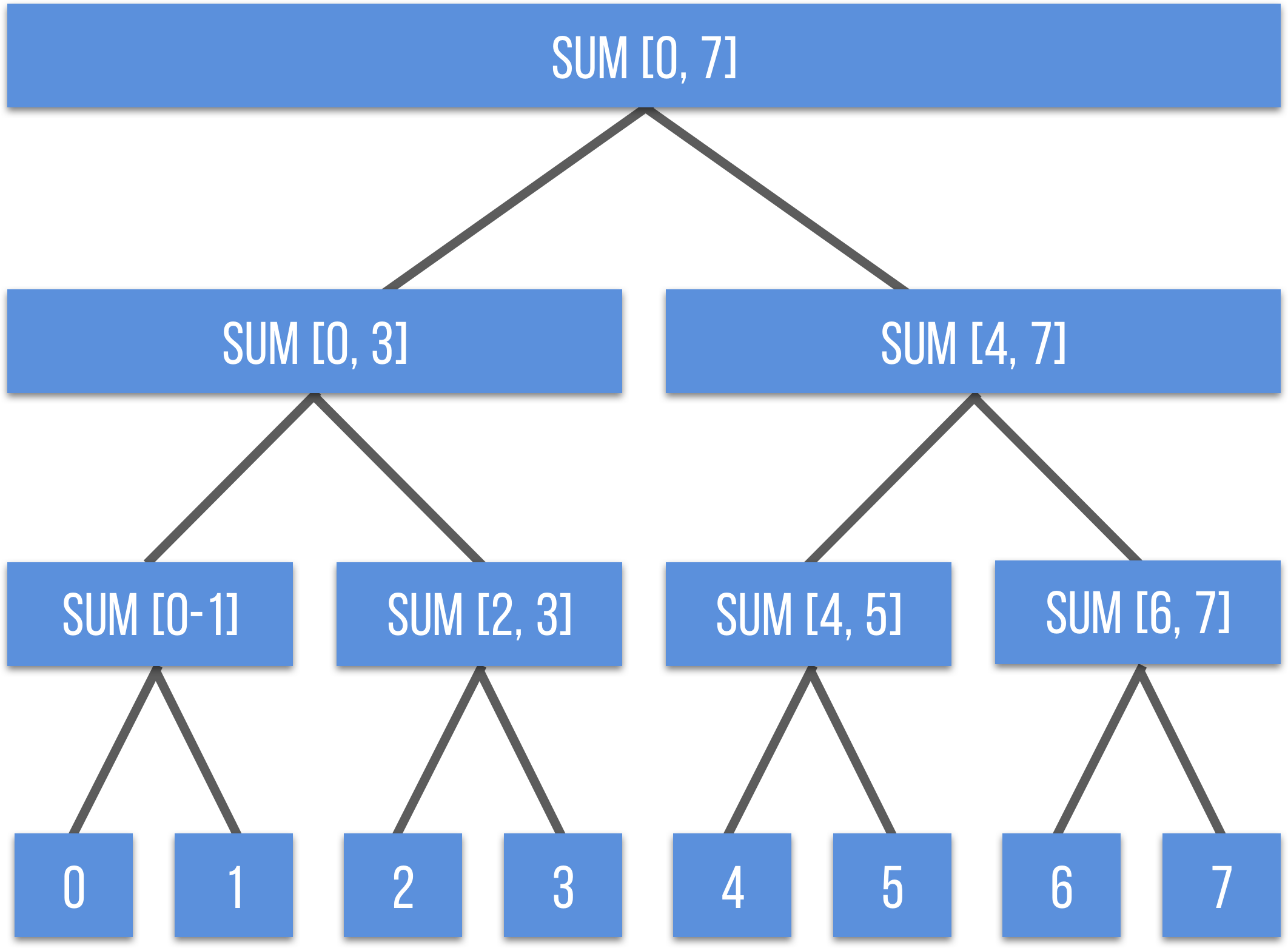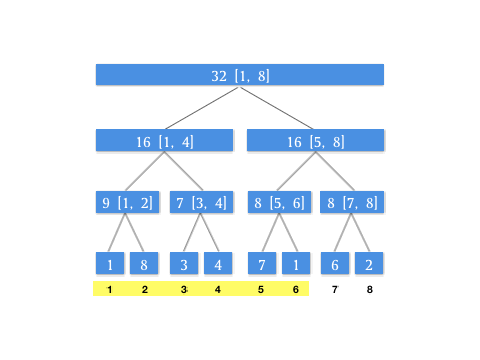# 用线段树再看 RMQ 问题GGTalk 播客

[28:30] 磊子：国内某特别爱招聘的大厂问过这么一道题：一个数组，要求得任意一个区间段内最大的数是多少。如果大家了解的话，就知道这题其实在考线段树….

# 线段树的概念# 不同场景下线段树的功能

## 求区间和

### 基础数据结构描述

$S = 2^H-1=2^{H-1}2-1=2N-1$

const int maxn = 1e4 + 7;

int tree[maxn << 2];


### Build 及 Push Up 操作

void push_up(int rt) {
tree[rt] = tree[rt << 1] + tree[rt << 1 | 1];
}/***
*
* @param l 当前节点描述范围的左边界
* @param r 当前节点描述范围的右边界
* @param rt 代表下标，tree[rt] 代表当前节点
*/
void build(int l, int r, int rt) {
if (l == r) {
tree[rt] = num[cur ++];
return ;
}
int m = (l + r) >> 1;
build(l, m, rt << 1);
build(m + 1, r, rt << 1 | 1);
// 这里递归到叶子执行一次向上更新
// 保证每一层都在上一层有数值之后执行
push_up(rt);
}


### Query 查询操作实现区间和/***
*
* @param L 待查询区间左边界
* @param R 待查询区间右边界
* @param l 当前节点描述范围的左边界
* @param r 当前节点描述范围的右边界
* @param rt 代表下标，tree[rt] 代表当前节点
*/
int query(int L, int R, int l, int r, int rt) {
if (L <= l && r <= R) {
return tree[rt];
}
int m = (l + r) >> 1;
int ret = 0;
if (L <= m) ret += query(L, R, l, m, rt << 1);
if (R > m)  ret += query(L, R, m + 1, r, rt << 1 | 1);
return ret;
}


### 单点更新操作

/***
*
* @param p 待更新的下标，指的在 num 中的下标
* @param replace 待替换的值
* @param l 当前节点描述范围的左边界
* @param r 当前节点描述范围的右边界
* @param rt 代表下标，tree[rt] 代表当前节点
*/
void update(int p, int replace, int l, int r, int rt) {
if (l == r) {
tree[rt] = replace;
return ;
}
int m = (l + r) >> 1;
if (p <= m) update(p, replace, l, m, rt << 1);
else update(p, replace, m + 1, r, rt << 1 | 1);
push_up(rt);
}


### 单元测试

#include <iostream>
#include <vector>
using namespace std;

const int maxn = 1e4 + 7;

vector<int> num(maxn);

int cur = 0;
int tree[maxn << 2];

void push_up(int rt) {
tree[rt] = tree[rt << 1] + tree[rt << 1 | 1];
}

/***
*
* @param l 当前节点描述范围的左边界
* @param r 当前节点描述范围的右边界
* @param rt 代表下标，tree[rt] 代表当前节点
*/
void build(int l, int r, int rt) {
if (l == r) {
tree[rt] = num[cur ++];
return ;
}
int m = (l + r) >> 1;
build(l, m, rt << 1);
build(m + 1, r, rt << 1 | 1);
push_up(rt);
}

/***
*
* @param L 待查询区间左边界
* @param R 待查询区间右边界
* @param l 当前节点描述范围的左边界
* @param r 当前节点描述范围的右边界
* @param rt 代表下标，tree[rt] 代表当前节点
*/
int query(int L, int R, int l, int r, int rt) {
if (L <= l && r <= R) {
return tree[rt];
}
int m = (l + r) >> 1;
int ret = 0;
if (L <= m) ret += query(L, R, l, m, rt << 1);
if (R > m)  ret += query(L, R, m + 1, r, rt << 1 | 1);
return ret;
}

/***
*
* @param p 待更新的下标，指的在 num 中的下标
* @param replace 待替换的值
* @param l 当前节点描述范围的左边界
* @param r 当前节点描述范围的右边界
* @param rt 代表下标，tree[rt] 代表当前节点
*/
void update(int p, int replace, int l, int r, int rt) {
if (l == r) {
tree[rt] = replace;
return ;
}
int m = (l + r) >> 1;
if (p <= m) update(p, replace, l, m, rt << 1);
else update(p, replace, m + 1, r, rt << 1 | 1);
push_up(rt);
}

int main() {
// [1, 8, 3, 4, 7, 1, 6, 2]
num = vector<int>({1, 8, 3, 4, 7, 1, 6, 2});
int n = num.size();
build(1, n, 1);
cout << query(1, 3, 1, n, 1) << endl; // 1 + 8 + 3 = 12
cout << query(3, 8, 1, n, 1) << endl; // 3 + 4 + 7 + 1 + 6 + 2 = 23
update(3, 10, 1, n, 1); // [1, 8, 10, 4, 7, 1, 6, 2]
cout << query(1, 3, 1, n, 1) << endl; // 1 + 8 + 10 = 19
}

/*
* 输出
* 12
* 23
* 19
*
*/


## RMQ 问题

void push_up(int rt) {
tree[rt] = max(tree[rt << 1], tree[rt << 1 | 1]);
}# 总结